1. Using GVB Orbitals in Physical Chemistry Courses

The familiar σB bonding and σA antibonding molecular orbitals (MOs) for H2 are shown here in 1D (axial) and 2D (contour cross-section) forms: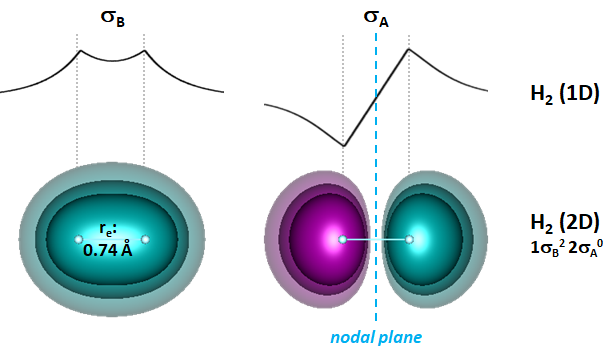Cyan and magenta coloring indicate positive and negative wavefunction amplitude, respectively. The bonding orbital exhibits the build-up of electronic charge between the nuclei that accounts for the bonding of the molecule, while the antibonding orbital has a node at the midpoint between the nuclei that excludes any overlap of electronic charge between two atoms.

To better understand the changes that occur when two hydrogen atoms come together to form H2, it would be useful to observe their orbitals over a range of separations instead of only at the equilibrium separation re of 0.74 Å. There is an immediate problem with doing this, however: the minimal antisymmetrized Hartree-Fock (HF) wavefunction built from the σB2 configuration and the singlet spin function [α(1)β(2)-β(1)α(2)] cannot dissociate into two hydrogen atoms.

Fortunately, there is a straightforward way to address this problem. We can expand the wavefunction by including the antibonding orbital:

Ψ = (cBσB2+cAσA2)(αβ-βα)

The coefficients cB and cA reflect the amount of each configuration that gives the best energy at a given internuclear separation. The HF wavefunction reduces to cB=1 and cA=0, which works fine near re but not at larger separations.

The figure shows the orbitals for this new H2 wavefuction at a number separations as well as the weights of the bonding and antibonding orbitals (the weights are the squares of the coefficients).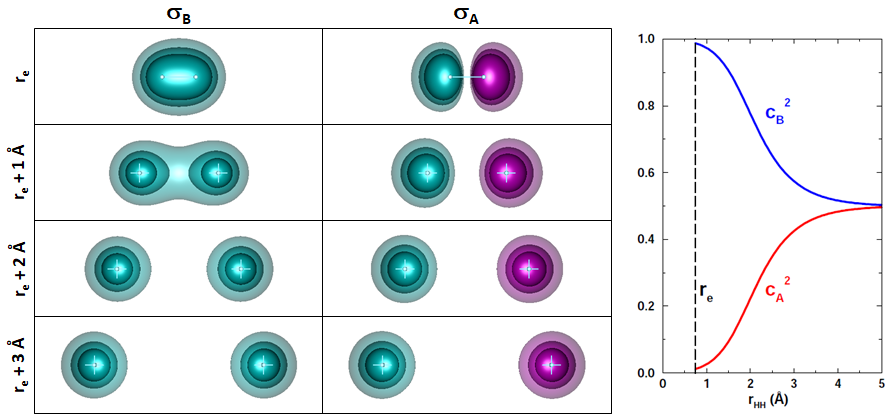The bonding orbital pulls apart into two pieces that become increasing spherical as the separation increases. The antibonding orbital is always two distinct pieces because there can be no overlap. The pieces distort away from each other at the miminum but they also become increasingly spherical as the separation increases. Note that the weights converge on the same value [SQRT(2)/2=0.7071] as r increases (after starting close to cB=1 and cA=0 at re).

While we see something similar to what we expect when the molecular orbitals are pulled apart, they nevertheless look wrong at large separations. We expect to see separate hydrogen atoms on the left and right, not half of each atom in both orbitals. Molecular orbitals tend to remain "molecular" even when it makes no intuitive sense for them to be that way.

The orbital pieces at r=3 Å suggest a way to fix the problem. If we add them together, the orbital on the left would gain amplitude while the pieces on the right cancel. If the antibonding orbital is subtracted from the bonding orbital, then the pieces on the left cancel. The orbitals in the top row of the next figure show the new orbitals, which finally look like two hydrogen atoms.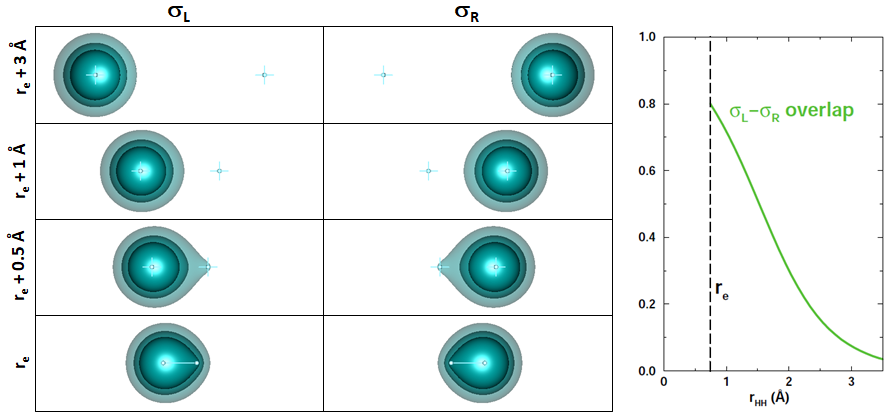The MOs from the other separations can also be tranformed. The math to do this involves simple algebraic expressions involving the coefficients cB and cA. No information is lost in making the transformation The new orbitals are known as generalized valence bond orbitals (GVBOs). Here's the math: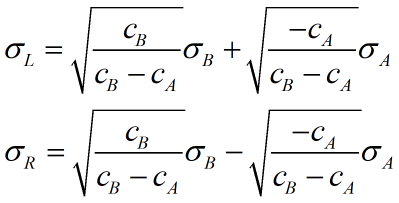As we follow the sequence above down from large separations to re, we see that the orbitals look like unaltered hydrogen atoms until they approach within about 1 Å of each other. Then each orbital polarizes or shifts toward the other nucleus as the electron responds to its attractive force. The orbitals can overlap in the region between the nuclei as long as the electrons have antiparallel spins (satisfying the Pauli principle). The overlap between the two GVBOs for H2 is shown in the graph on the right. It increases from zero at long separations to about 0.8 at re. Here's the simple math for calculating the GVB overlap S from the coefficients cB and cA: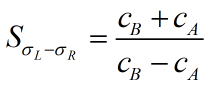We've seen that when the spins of the two hydrogen electrons are antiparallel, the electrons can overlap and maximize the electron-proton attraction that makes H2 a stable, bound molecule. Since two electrons with opposite spins yields a singlet, we can think of the bond forming due to singlet coupling the electrons together. Of course, the interaction between the two electrons is always repulsive. The coupling diagram for H2 from the two atoms can be depicted as: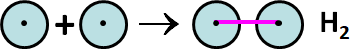If the electrons have parallel spins, the net spin is a triplet. No bond forms between two hydrogen atoms with the same spin because their orbitals are forbidden from overlapping by the Pauli principle.

On this page, we've only seen static images of orbitals. The next section shows animations of the GVBOs for H2 and other molecules.